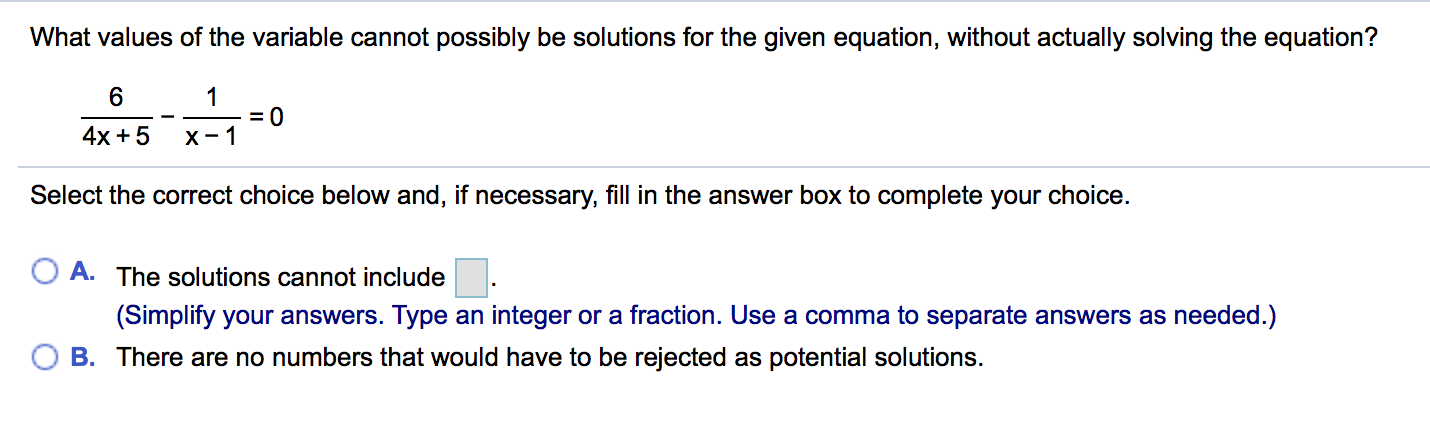# What values of the variable cannot possibly be solutions for the given equation, without actually solving the equation?64x+5 x-1Select the correct choice below and, if necessary, fill in the answer box to complete your choice.O A. The solutions cannot includeO B. There are no numbers that would have to be rejected as potential solutions.(Simplify your answers. Type an integer or a fraction. Use a comma to separate answers as needed.)

Question
12 viewshelp_outlineImage TranscriptioncloseWhat values of the variable cannot possibly be solutions for the given equation, without actually solving the equation? 6 4x+5 x-1 Select the correct choice below and, if necessary, fill in the answer box to complete your choice. O A. The solutions cannot include O B. There are no numbers that would have to be rejected as potential solutions. (Simplify your answers. Type an integer or a fraction. Use a comma to separate answers as needed.) fullscreen
check_circle

Step 1

The values which cannot be the solutions of the given equation has to be evaluated without solving the following equation,

Step 2

We can determine those values by actually not solving the entire equation ib the following manner,

Since the equation is in fraction form, therefore those values which makes the denominator ze...

### Want to see the full answer?

See Solution

#### Want to see this answer and more?

Solutions are written by subject experts who are available 24/7. Questions are typically answered within 1 hour.*

See Solution
*Response times may vary by subject and question.
Tagged in

### Algebra